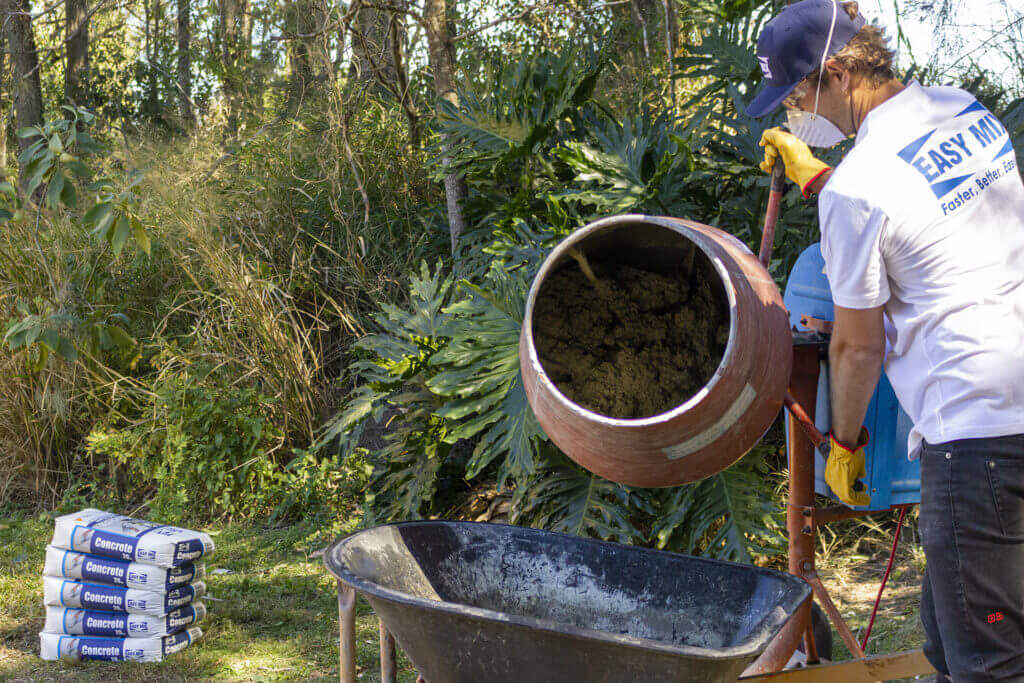# How much concrete do you need?Are you wondering how much concrete you need to complete your backyard project? We’ve written this handy guide to help you figure out the number of Easy Mix premixed bags you’ll need to purchase.

Before we get into our guide; if maths is not your thing this link will take you to a concrete calculator that will do all the hard work for you. Just remember to have all your measurements ready beside you.

Now for those of you who are maths enthusiasts, let’s dive in.

### Laying a concrete slab

This calculation will help you determine the amount of concrete needed for a rectangular or square slab.

1. Measure the length, width and depth of the area that will be filled with concrete. Make sure your measurements are in metres, this will make things easier.
2. Calculate the total m3 of the area.

Use this formula: length x width x depth

We know that 1 x 20kg bag of cement makes 0.01m3 of concrete, and that 100 x 20kg bags of cement make 1m3 of concrete.

1. Now we calculate the number of bags we need.

Use this formula: total m3 of the area x 100

Let’s run through a little example with these measurements: 1.5m long, 0.5m width and 0.075m deep.

Total m3 of the area = 1.5 x 0.5 x 0.075 = 0.05625m3

Number of bags needed = 0.05625 x 100 = 5.625 bags. Let’s round up to 6 bags.

If you have a shape that’s not a rectangle or square, we recommend using this concrete calculator to figure out how much concrete you will need for your project.

### Installing posts

There are a couple more steps to determining the amount of concrete needed to install posts. We’re going to calculate the amount needed for 1 post, remember at the end to multiply the number of bags by the total number of posts you will be installing.

1. Measure the height and width of the post. Make sure your measurements are in metres.
2. Calculate the depth of the hole required for your post.

Use this formula: (post height ÷ 3) + 0.1m

1. Next calculate the m3 of the post section which will be in the hole.

Use this formula: width of post x width of post x depth of hole

1. Next calculate the m3 of the hole

Use this formula: (width of post x 2)2 x depth of hole

1. Now calculate the total m3 of the area

Use this formula: m3 of hole – m3 of post section

We know that 1 x 20kg bag of cement makes 0.01m3 of concrete, and that 100 x 20kg bags of cement make 1m3 of concrete.

1. Now we calculate the number of bags we need.

Let’s run through a little example with these measurements: post height is 1.8m and the post width is 0.075m.

Depth of the hole = (1.8 ÷ 3) + 0.1 = 0.7m

m3 of the post section = 0.075 x 0.075 x 0.7 = 0.00393m3

m3 of the hole = (0.075 x 2)2 x 0.7 = 0.01575m3

Total m3 of the area = 0.01575 – 0.00393 = 0.0118m3 = approx. 0.012m3

If 1 bag makes 0.01m3, then for 0.012m3 it’s best to use 1.5 bags.

Remember to count the number of posts and multiple it by the number of bags required for installing one post.

Now you know how many bags you will need for your project, you are ready to begin. Need some guidance on the steps to lay concrete or set a post, check out our simple how-to-guides.

## Laying and Filling Pavers

Laying and filling pavers is easy with the Easy Mix range of landscaping sand and gravel products. Before You Start…